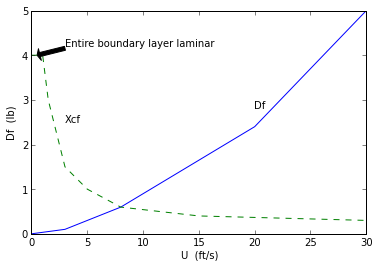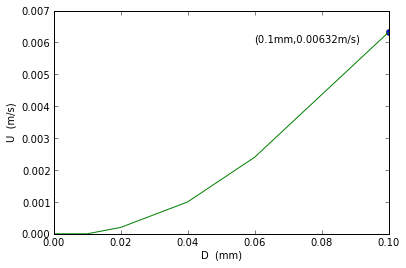Chapter 9:Flow over immersed bodies¶

Example 9.1 Page no.487¶

In :
U=25.0                     #ft/sec
p=0                        #gage
b=10.0                     #ft
t=1.24*(10**-3)            #where t=stress*(x**0.5)
a=0.744                    #where a=p/(1-((y**2)/4))
p1=-0.893                  #lb/(ft**2)
from scipy import integrate
def f(x):
return(2*t*b/(x**0.5))
print "The drag when plate is parallel to the upstream flow=",drag1,"lb"

def f1(y):
return((((a*(1-((y**2)/4))))-p1)*b)

#result
print  "The drag when plate is perpendicular to the upstream flow=",drag2,"lb"
The drag when plate is parallel to the upstream flow= 0.0992 lb
The drag when plate is perpendicular to the upstream flow= 55.56 lb

Example 9.5 Page no.508¶

In :
U=10.0                        #ft/sec
Twater=60.0                #degree F
Tglycerin=68.0            #degree F
kviswater=1.21*(10**-5)          #(ft**2)/sec
kvisair=1.57*(10**-4)               #(ft**2)/sec
kvisglycerin=1.28*(10**-2)       #(ft**2)/sec
Re=5*(10**5)                         #assumption

#calculation
xcrwater=kviswater*Re/U          #ft
xcrair=kvisair*Re/U                   #ft
xcrglycerin=kvisglycerin*Re/U   #ft
btwater=5*(kviswater*xcrwater/U)**0.5       #ft where bt=thickness of boundary layer
btair=5*(kvisair*xcrair/U)**0.5                             #ft
btglycerin=5*(kvisglycerin*xcrglycerin/U)**0.5     #ft

#result
print "a)WATER"
print "location at which boundary layer becomes turbulent=",round(xcrwater,2),"ft"
print "Thickness of the boundary layer=",round(btwater,5),"ft"
print "b)AIR"
print "location at which boundary layer becomes turbulent=",round(xcrair,2),"ft"
print "Thickness of the boundary layer=",round(btair,3),"ft"
print "c)GLYCERIN"
print "location at which boundary layer becomes turbulent=",round(xcrglycerin,2),"ft"
print "Thickness of the boundary layer=",round(btglycerin,2),"ft"
a)WATER
location at which boundary layer becomes turbulent= 0.61 ft
Thickness of the boundary layer= 0.00428 ft
b)AIR
location at which boundary layer becomes turbulent= 7.85 ft
Thickness of the boundary layer= 0.056 ft
c)GLYCERIN
location at which boundary layer becomes turbulent= 640.0 ft
Thickness of the boundary layer= 4.53 ft

Example 9.7 Page no.512¶

In :
%matplotlib inline
Welcome to pylab, a matplotlib-based Python environment [backend: module://IPython.zmq.pylab.backend_inline].
In :
T=70.0        #degree F
U1=0.0        #ft/sec
U2=30.0       #ft/sec
l=4.0         #ft
b=0.5         #ft
d=1.94
vis=2.04*(10**(-5))
x=d*l/vis
U=10.0       #ft/s  U1 < U < U2

#calculation
import math
Re=d*l*U/(vis)    #Reynold no.
#but we take Re approximately =3800000
Re_=3.8*10**6
Cd=0.455/((math.log10(Re_))**2.58)-(1700.0/Re_)
D=d*U**2*Cd
print "The Drag is ",round(D,3),"lb"

#Plot
import numpy as np
import matplotlib.pyplot as plt
fig = plt.figure()

U=[0,3,8,20,30]
Df=[0,0.1,0.598,2.4,5]
xlabel("U  (ft/s)")
ylabel("Df  (lb)")
plt.xlim((0,30))
plt.ylim((0,5))
a=plot(U,Df)

U1=[0,1,1.5,3,5,8,15,30]
Df1=[4,4,3,1.5,1,0.598,0.4,0.3]
ax.annotate('Entire boundary layer laminar', xy=(0.5, 4), xytext=(3,4.2),
arrowprops=dict(facecolor='black', shrink=0.001),
)
xlabel("U  (ft/s)")
ylabel("Df  (lb)")
plt.xlim((0,30))
plt.ylim((0,5))

a=plot(U1,Df1,linestyle='--')
plt.text(20,2.8,'Df')
plt.text(3,2.5,'Xcf')

show(a)
The Drag is  0.597 lbExample 9.10 Page no.525¶

In :
D=0.1                      #mm
sg=2.3
vis=1.12*(10**(-3))    #N*s/(m**2)
#by free body diagram and assuming CD=24/Re
U=(sg-1)*999*9.81*((D/1000)**2)/(18*vis)

#Result
print "The velocity of the particle through still water =",round(U,3),"m/s"

#Plot
import matplotlib.pyplot as plt
fig = plt.figure()

D=[0,0.01,0.02,0.04,0.06,0.1]
U=[0,0,0.0002,0.001,0.0024,0.00632]
xlabel("D  (mm)")
ylabel("U  (m/s)")
plt.xlim((0,0.1))
plt.ylim((0,0.007))

ax.plot([0.1], [0.00632], 'o')
ax.annotate('(0.1mm,0.00632m/s)', xy=(0.06,0.006))
a=plot(D,U)
show(a)
The velocity of the particle through still water = 0.006 m/sExample 9.11 Page no.528¶

In :
D=1.5                     #in

CD=0.5
dice=1.84                  #slugs/(ft**3) density of ice
dair=2.38*(10**(-3))  #slugs/(ft**3)
U=(4*dice*32.2*(D/12)/(3*dair*CD))**0.5     #ft/sec

#Result
print "The velocity of the updraft needed=",round(U*3600/5275,1),"mph"
The velocity of the updraft needed= 62.2 mph

Example 9.12 Page no.531¶

In :
Dg=1.69                    #in.
Wg=0.0992               #lb
Ug=200                     #ft/sec
Dt=1.5                       #in.
Wt=0.00551               #lb
Ut=60                            #ft/sec
kvis=(1.57*(10**(-4)))    #(ft**2)/sec

#Calculation
import math
Reg=Ug*Dg/kvis
Ret=Ut*Dt/kvis
#the corresponding drag coefficients are calculated as
CDgs=0.25                   #standard golf ball
CDgsm=0.51                #smooth golf ball
CDt=0.5                       #table tennis ball
Dgs=0.5*0.00238*(Ug**2)*math.pi*((Dg/12)**2)*CDgs/4          #lb
Dgsm=0.5*0.00238*(Ug**2)*math.pi*((Dg/12)**2)*CDgsm/4     #lb
Dt=0.5*0.00238*(Ut**2)*math.pi*((Dt/12)**2)*CDt/4                 #lb
#the  corresponding decelerations are a=D/s=g*D/W
#deceleration relative to g=D/W
decgs=Dgs/Wg
decgsm=Dgsm/Wg
dect=Dt/Wt

#result
print"STANDARD GOLF BALL:"
print "The drag coefficient=",round(Dgs,3),"lb"
print "The deceleration relative to g=",round(decgs,2)
print"SMOOTH GOLF BALL:"
print "The drag coefficient=",round(Dgsm,3),"lb"
print"The deceleration relative to g=",round(decgsm,2)
print"TABLE TENNIS BALL:"
print "The drag coefficient=",round(Dt,3),"lb"
print "The deceleration relative to g=",round(dect,2)
STANDARD GOLF BALL:
The drag coefficient= 0.185 lb
The deceleration relative to g= 1.87
SMOOTH GOLF BALL:
The drag coefficient= 0.378 lb
The deceleration relative to g= 3.81
TABLE TENNIS BALL:
The drag coefficient= 0.026 lb
The deceleration relative to g= 4.77

Example 9.13 Page no.533¶

In :
U=88                   #fps
Ds=40.0                #ft
Dc=15.0                #ft
b=50#ft
Res=U*Ds/(1.57*(10**(-4)))
Rec=U*Dc/(1.57*(10**(-4)))

#calculation
import math
#from these values of Re drag coefficients are found as
CDs=0.3
CDc=0.7
#by summing moments about the base of the tower
Drs=0.5*0.00238*(U**2)*math.pi*(Ds**2)*CDs/4     #lb
Drc=0.5*0.00238*(U**2)*b*Dc*CDc                   #lb
M=(Drs*(b+(Ds/2)))+(Drc*(b/2))                          #ft*lb

#Result
print "The moment needed to prevent the tower from tripping=",round(M,3),"lb*ft"
The moment needed to prevent the tower from tripping= 364139.221 lb*ft

Example 9.15 Page no.544¶

In :
U=15                   #ft/sec
b=96                    #ft
c=7.5                    #ft
W=210                 #lb
CD=0.046
eff=0.8                  #power train efficiency
d=2.38*(10**(-3))       #slugs/(ft**3)
#W=L
CL=2*W/(d*(U**2)*b*c)
D=0.5*d*(U**2)*b*c*CD
P=D*U/(eff*550)       #hp

#Result
print "The lift coefficient=",round(CL,3)
print "The power required by the pilot=",round(P,3),"hp"
The lift coefficient= 1.089
The power required by the pilot= 0.302 hp

Example 9.16 Page no.548¶

In :
W=2.45*(10**(-2))               #N
D=3.8*(10**(-2))                  #m
U=12                                    #m/s

#calculation
import math
#W=L
d=1.23                                 #kg/(m**3)
W=0.5*d*(U**2)*(D**2)*math.pi*CL/4
CL=2*W/(d*(U**2)*math.pi*(D**2)/4)
#using this value of CL, omega*D/(2*U)=x is found as
x=0.9